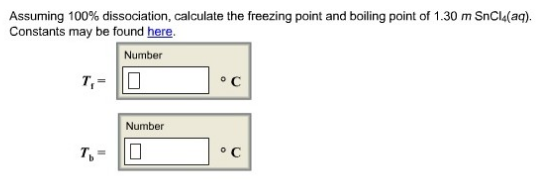Chemistry Practice Problems Freezing Point Depression Practice Problems Solution: Assuming 100% dissociation, calculate the freezing...

🤓 Based on our data, we think this question is relevant for Professor Bindell's class at UCF.

# Solution: Assuming 100% dissociation, calculate the freezing point and boiling point of 1.30 m SnCl4 (aq). Constants may be found here.

###### Problem

Assuming 100% dissociation, calculate the freezing point and boiling point of 1.30 m SnCl(aq). Constants may be found here.View Complete Written Solution

Freezing Point Depression

Freezing Point Depression

#### Q. What is the change in freezing point temperature (ΔTf) of water if 0.25 m of Glucose is present in solution (Kf = 1.86°C/m)?a. 1.86°Cb. 1.00°Cc. 0.465...

Solved • Tue Jun 26 2018 12:34:10 GMT-0400 (EDT)

Freezing Point Depression

#### Q. Which of the solution has the highest freezing point?

Solved • Mon Jun 25 2018 15:33:13 GMT-0400 (EDT)

Freezing Point Depression

#### Q. How many grams of ethylene glycol, C 2H4(OH)2, must be added to 400.0 g of water to yield a solution that will freeze at -8.35 °C?a) 37 gb) 111 gc) 75...

Solved • Mon Jun 25 2018 14:34:17 GMT-0400 (EDT)

Freezing Point Depression

#### Q. Part CCalculate the freezing point of a solution containing 3.5 % KCl by mass (in water). Express your answer using two significant figures. Part DCal...

Solved • Wed Jun 06 2018 14:34:52 GMT-0400 (EDT)